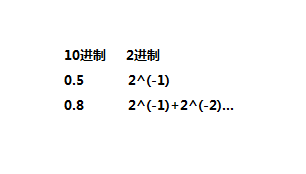# 燕十八PHP基础

## 变量

#### 变量检测

1. bool isset ( mixed $var [, mixed$… ] )— 检测变量是否设置
注意：一次可以检测多个变量，有一个变量未设置，则返回false; 如果 var 存在并且值不是 NULL 则返回 TRUE，否则返回 FALSE。

1. string gettype ( mixed $var ) — 获取变量的类型 返回值： “boolean” “integer” “double”（由于历史原因，如果是 float 则返回“double”，而不是“float”） “string” “array” “object” “resource” 判断是否是指定类型的变量：is_array,is_bool,is_float（is_double）,is_int（is_integer）,is_null,is_string,is_object,is_resource #### 变量类型转换 最常见的是字符串与数字的转换，或是数字/字符串->布尔值的转换。 $a='12avaf1422';
$b=1; echo$a+$b;//13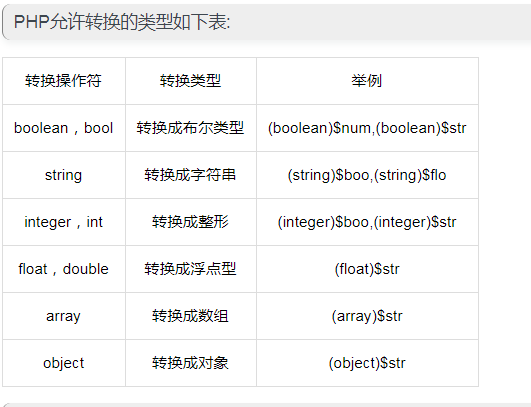$num1=3.14;
$num2=(int)$num1;
var_dump($num1); echo "<br/>"; var_dump($num2);//int(3)

1. empty() — 检查一个变量是否为空 当var存在，并且是一个非空非零的值时返回 FALSE 否则返回 TRUE.
以下的东西被认为是空的：
“” (空字符串)
0 (作为整数的0)
0.0 (作为浮点数的0)
“0” (作为字符串的0)
NULL(null值)
FALSE（布尔值false）
array() (一个空数组)
$var; (一个声明了，但是没有值的变量) #### 传值赋值与引用赋值 $num1=1;
$num2=2; echo$num2;//2
$num2=&$num1;
echo $num2;//1  引用赋值结合变量销毁unset()： $num1=0;
$num2=&$num1;
unset($num1); var_dump($num2,$num1);//int(0) NULL  注意：$num1销毁变量后，$num2值依然存在 #### 动态变量名 $mk='孟珂';
$$mk='裴丹圣'; echo mk,'love',$$mk;


## 常量

### 定义

• 该值在脚本中不能改变，不能销毁，不能重新声明
• 常量名不需要加 $修饰符 • 常量名称习惯由大写字母组成，便于与变量区分；用define定义：bool define ( string$name , mixed $value [, bool$case_insensitive = false ] )，case_insensitive ：可选参数，如果设置为 TRUE，该常量则大小写不敏感。默认是大小写敏感的
• 用defined（）检测常量是否存在

#### 输出

define('PRICE',100);

echo PRICE;//100


#### 动态常量名

constant()

define('PI',3.14);
$chang='PI'; echo constant($chang);


## 运算符

#### 算数运算符

var_dump(100000000000000000000000);//float(1.0E+23)


$a=-10;//换成10试试$b=3;//换成-3试试
echo $a%$b;


#### 比较运算符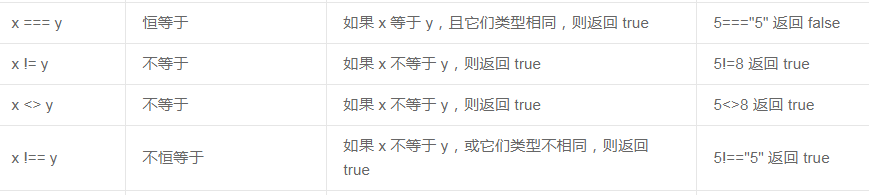#### 三元运算符

(expr1) ? (expr2) : (expr3)

$test=1;$re=$test??'不存在';//PHP7+写法$re=$test?:'不存在';// PHP 5.3+普通写法 echo$re,PHP_EOL;
echo 'hi';


#### 逻辑运算符

x xor y 异或 如果 x 和 y 有且仅有一个为 true，则返回 true

echo( (10 xor 1) ?:'假');//假

1. 关于“短路”
// foo() 根本没机会被调用，被运算符“短路”了

$a = (false && foo());$b = (true  || foo());
$c = (false and foo());$d = (true  or  foo());

1. 关于运算符优先级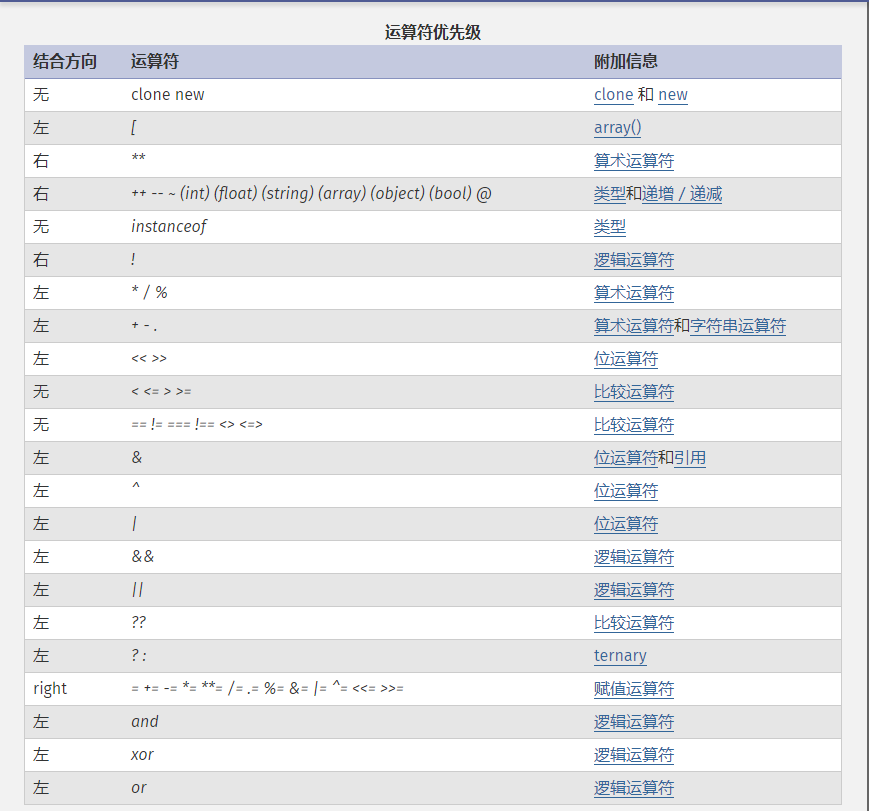“||” 比 “or” 的优先级高
“&&” 比 “and” 的优先级高
=比 and or 的优先级高
$e = false || true;$f = false or true;
var_dump($e,$f);//true false
$g = true && false;$h = true and false;
var_dump($g,$h);//false  true


#### 递增递减运算符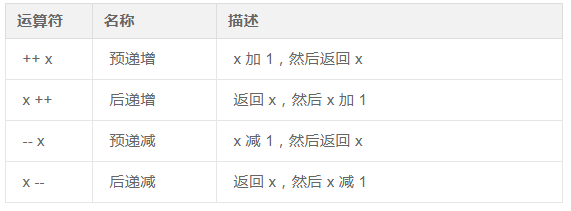.就一个点，我只说一次！

#### 赋值运算符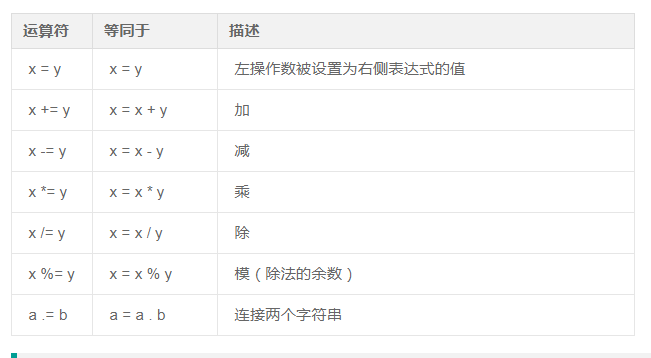## 控制结构

#### do while

do
{

}
while (条件);


#### for

for (初始值; 条件; 增量)
{
要执行的代码;
}


#### break 和continue 区别

break 在循环中作用是结束一个循环
continue立即结束本次循环，继续执行下一次循环

## 函数

PHP 的真正威力源自于它的函数！

#### 函数传参方式

function t($a) {$a += 1;
return $a; }$a = 1;
t($a); echo$a;//输出1


function t(&$a) {$a += 2;
return $a; }$b = 1;
t($b); echo$b;//输出3


#### 函数作用域

global 关键字用于函数内访问全局变量。

$x=5;$y=10;

function myTest()
{
global $x,$y;
$y=$x+$y; } myTest(); echo$y; //15;


PHP将所有全局变量存储在一个名为$GLOBALS[index]的数组中。index为 保存变量的名称。上面的实例可以写成这样： $x=5;
$y=10; function myTest() {$GLOBALS['y']=$GLOBALS['x']+$GLOBALS['y'];
}

myTest();
$heart();  #### 时间戳函数 time(); 返回当前时间戳 microtime(get_as_float) 函数返回当前 Unix 时间戳的微秒数。 如果 get_as_float 参数设置为 TRUE，则返回浮点数，表示自 Unix 纪元(January 1 1970 00:00:00 格林威治时间（GMT）)起精确到微秒的以秒为单位的当前时间. echo microtime();//0.68480900 1539325171 echo '<hr>'; echo microtime(true);//1539325171.6848  可在程序开头和结尾分别放一个，两者相减，用于计算程序运行时间。 #### 时间戳格式化 echo date('Y-m-d,星期l,a h:i:s');//2018-10-12,星期Friday,AM 06:43:21 echo gmdate('Y-m-d,星期l,a h:i:s');//2018-10-12,星期Friday,AM 06:43:21  date(format,timestamp) gmdate(format,timestamp) timestamp 可选。规定时间戳。。默认是当前时间和日期 两个函数日期都是自GMT起。 PHP 5.1.0：有效范围的时间戳是从 1901 年 12 月 13 日 20:45:54 GMT 星期五 到 2038 年 1 月 19 日 03:14:07 GMT 星期二。5.1.0 之前的版本，在某些系统上（例如 Windows）时间戳被限制在从 01-01-1970 到 19-01-2038。 #### 日期解析函数 mktime(hour,minute,second,month,day,year) 函数返回日期的 Unix 时间戳。Unix 时间戳包含 Unix 纪元（1970 年 1 月 1 日 00:00:00 GMT）与指定时间之间的秒数。 strtotime() 函数用于把人类可读的字符串转换为 Unix 时间。 strtotime(' today+1day')  checkdate(month,day,year);如果日期是有效的则返回 TRUE，否则返回 FALSE。 #### Math函数 • abs(x) 返回一个数的绝对值。 • ceil(x) 向上舍入为最接近的整数。 返回的类型仍然是 float，因为 float 值的范围通常比 integer 要大。 • floor(): 去尾法取整 • fmod(): 浮点数取余 • pow(): 返回数的n次方 • round(): 浮点数四舍五入 • max(): 求最大值 注释：如果仅有一个参数且为数组，max() 返回该数组中最大的值。如果第一个参数是整数、字符串或浮点数，则至少需要两个参数而 max() 会返回这些值中最大的一个。可以比较无限多个值。 echo max(1, 3, 5, 6, 7);//7 echo max(array(2, 4, 5));//5  • trim(string,charlist) 移除字符串两侧的空白字符或其他预定义字符。 $str = "Hello World!";
echo $str . "<br>";//Hello World! echo trim($str,"Hled!");//o Wor

• ltrim() - 移除字符串左侧的空白字符或其他预定义字符
• rtrim() - 移除字符串右侧的空白字符或其他预定义字符

#### Filesystem函数

• dirname(path)返回路径中的目录部分

## 字符串

#### 字符串定义方式

heredoc,nowdoc

//heredoc
$a='孟珂';$str1=<<<INTRO
hello world
{$a}you ok? INTRO; echo$str1;

echo $str1;//hello world 孟珂you ok? //nowdoc$a='孟珂';
$str1=<<<'INTRO' hello world {$a}you ok?
INTRO;
echo $str1;//hello world {$a}you ok?


• 相同点：用于定义大段文本；
1.标识符可以自定义 一般的 有EOT ，EOD EOF 等， 只有保持开始表示符和结束表示符一样即可。
2.结束表示符必须独占一行，且必须顶格写，不能有空格，最后以 ‘;’ 分号结尾。
3.所有引用的字符串中可以包含变量，无需字符串连接符。
• 不同点：（同单引号和双引号的不同点）
转义
变量解析
速度：单引号不需要分析串内有没有变量，需要转义的内容也少，速度更快。

#### 字符串常用函数

strlen(string) 函数返回字符串的长度。
utf-8编码，一个中文占3个字节
mixed mb_strlen ( string $str [, string$encoding = mb_internal_encoding() ] )

encoding 参数为字符编码。如果省略，则使用内部字符编码。

echo mb_strlen('孟珂','utf-8');//2


strpos(string,find,start)

• 对大小写敏感。
• 如果没有找到字符串则返回 FALSE。
• 注释： 字符串位置从 0 开始，不是从 1 开始。
1. stripos() - 查找字符串在另一字符串中第一次出现的位置（不区分大小写）
2. strripos() - 查找字符串在另一字符串中最后一次出现的位置（不区分大小写）
3. strrpos() - 查找字符串在另一字符串中最后一次出现的位置（区分大小写）

str_replace(find,replace,string,count)str_replace(find,replace,string,count)

str_ireplace() 函数执行不区分大小写的搜索。

echo str_replace('fuck','**','fuckyoufuck');//**you**

• 如果搜索的字符串是数组，那么它将返回数组。
• 如果搜索的字符串是数组，那么它将对数组中的每个元素进行查找和替换。
• 如果查找的是数组，而替换的是字符串，那么替代字符串将对所有查找到的值起作用。
print_r(str_replace('fuck','***',array('fuck you','fuckyoufuck')));//Array (  => *** you  => ***you*** )

• 如果同时需要对数组进行查找和替换，并且需要执行替换的元素少于查找到的元素的数量，那么多余元素将用空字符串进行替换
$find = array("Hello","world");$replace = array("B");
$arr = array("Hello","world","!"); print_r(str_replace($find,$replace,$arr));//Array (  => B  =>  => ! )


$find = array("Hello","world");$replace = array("B",'c');
$arr = array("Hello","world","!"); print_r(str_replace($find,$replace,$arr));//Array (  => B  => c  => ! )


strtr(string,from,to)或strtr(string,array) 批量替换字符串

echo strtr('hello world',['hello'=>'hi','world'=>'earth']);//hi earth


substr(string,start[,length])

length可选。规定被返回字符串的长度。默认是直到字符串的结尾
explode(separator,string,limit)

implode(separator,array)

separator 可选。规定数组元素之间放置的内容。默认是 “”（空字符串）

• str_pad(string,length,pad_string,pad_type)
把字符串填充为新的长度。
$str = "Hello World"; echo str_pad($str,30,".");//Hello World...................


• str_repeat(string,repeat)
返回值： 返回被重复的字符串。
• str_split(string,length)
把字符串分割到数组中。
返回值： 返回分割后的数组。
如果 length 小于 1，则 str_split() 函数将返回 FALSE。
如果 length 大于字符串的长度，则整个字符串将作为数组的唯一元素返回。
注释：length 可选。规定每个数组元素的长度。默认是 1。

## 数组

#### 声明方式

1. array()来声明；
2. 是一种复合数据，可以装下多个值，每个值用不同的键来区分。

#### 数组类型

1. 索引数组：键五特殊意义，为纯数字
2. 关联数组 ：字符串做键，一般能体现该单元的内容
3. 多维数组

#### 数组键规则

1. 如果不声明键，则从0，1,2递增来生成键

2. 如果已经存在某1个或几个数字键，则从最大的数字键，递增生成数字键

$arr=array(3=>'a','b'); print_r($arr);//Array (  => a  => b )

1. 如果键声明重复了，后面的值覆盖前面的值
$arr=array(3=>'a',3=>'b','c'); print_r($arr);//Array (  => b  => c )


#### 操作数组单元

• 增加单元
$arr[]=$value;
或者：
$arr[$key]=$value; • 读取单元值$arr[$key] • 修改单元值$arr[$key]=$value
• 删除单元值
unset[$arr[$key]]

#### 遍历数组

foreach($arr as$value){}

foreach($arr as$key=>$value){} #### 游标操作 • current() 函数返回数组中的当前元素的值。 • end() - 将内部指针指向数组中的最后一个元素，并输出 • next() - 将内部指针指向数组中的下一个元素，并输出 • prev() - 将内部指针指向数组中的上一个元素，并输出 • reset() -将内部指针指向数组中的第一个元素，并输出 • each() - 返回当前元素的键名和键值，并将内部指针向前移动 each() 函数在 PHP 7.2.0 中被弃用了。 #### 数组常用函数 • array_push(array,value1,value2…) 函数向第一个参数的数组尾部添加一个或多个元素（入栈）， 返回值： 返回新数组的长度。 • 注释：即使数组中有字符串键名，您添加的元素也始终是数字键 • 注释：如果用 array_push() 来给数组增加一个单元，还不如用$array[] =，因为这样没有调用函数的额外负担
• 注释：如果第一个参数不是数组，array_push() 将发出一条警告。这和$var[] 的行为不同，后者会新建一个数组。 • array_pop(array) 函数删除数组中的最后一个元素。 返回值： 返回数组的最后一个值。如果数组是空的，或者非数组，将返回 NULL。 • array_unshift(array,value1,value2,value3…) 用于向数组插入新元素。新数组的值将被插入到数组的开头。 返回值： 返回数组中新的元素数目。 • array_shift(array) 删除数组中第一个元素 返回值： 返回被删除元素的值，如果数组为空则返回 NULL • array_key_exists(key,array) 检查某个数组中是否存在指定的键名 返回值： 如果键名存在则返回 true，如果键名不存在则返回 false。 • in_array(search,array,type) 搜索数组中是否存在指定的值。 返回值：如果给定的值 search 存在于数组 array 中则返回 true。如果第三个参数设置为 true，函数只有在元素存在于数组中且数据类型与给定值相同时才返回 true。 如果没有在数组中找到参数，函数返回 false。 注释：如果 search 参数是字符串，且 type 参数设置为 true，则搜索区分大小写。 • array_merge(array1,array2,array3…) 把一个或多个数组合并为一个数组。 返回值： 返回合并的数组。 注释：如果两个或更多个数组元素有相同的键名，则最后的元素会覆盖其他元素。 注释：如果您仅向 array_merge() 函数输入一个数组，且键名是整数，则该函数将返回带有整数键名的新数组，其键名以 0 开始进行重新索引。 $a=array(3=>"red",4=>"green");
print_r(array_merge($a)); //Array (  => red  => green )  • array_merge_recursive(array1,array2,array3…) 把一个或多个数组合并为一个数组。 返回值： 返回合并的数组。 注释： 该函数与 array_merge() 函数的区别在于处理两个或更多个数组元素有相同的键名时。array_merge_recursive() 不会进行键名覆盖，而是将多个相同键名的值递归组成一个数组。 注释： 如果您仅仅向 array_merge_recursive() 函数输入一个数组，结果与 array_merge() 相同，函数将返回带有整数键名的新数组，其键名以 0 开始进行重新索引。 $a1=array("a"=>"red","b"=>"green");
$a2=array("c"=>"blue","b"=>"yellow"); print_r(array_merge_recursive($a1,$a2)); //Array ( [a] => red [b] => Array (  => green  => yellow ) [c] => blue )  • sort(array,sortingtype); 对索引数组按键值进行升序排序。 返回值：如果成功则返回 TRUE，否则返回 FALSE。 注释：本函数为数组中的单元赋予新的键名。原有的键名将被删除。 $cars=array('v'=>"Volvo",'b'=>"BMW",'t'=>"Toyota");
sort($cars); var_dump($cars);
//array(3) {
=>
string(3) "BMW"
=>
string(6) "Toyota"
=>
string(5) "Volvo"
}

• rsort(array,sortingtype);
对数值(即索引)数组按键值进行降序排序。
返回值：如果成功则返回 TRUE，否则返回 FALSE。
• usort(array,myfunction);
通过用户自定义的比较函数对数组进行排序。
返回值： 若成功则返回 TRUE，若失败则返回 FALSE。
function my_sort($a,$b)
{
if ($a==$b) return 0;
return ($a<$b)?-1:1;
}

$a=array(4,2,8,6); usort($a,"my_sort");
print_r($a);//Array (  => 2  => 4  => 6  => 8 )  • asort(array,sortingtype); 对关联数组按照键值进行升序排序。 返回值： 如果成功则返回 TRUE，如果失败则返回 FALSE。 • arsort() 函数对关联数组按照键值进行降序排序。 • ksort() 函数对关联数组按照键名进行升序排序。 • krsort(array,sortingtype); 对关联数组按照键名进行降序排序。 • array_diff(array1,array2,array3…); 返回两个数组的差集数组。 返回值： 返回差集数组，该数组包括了所有在被比较的数组（array1）中，但是不在任何其他参数数组（array2 或 array3 等等）中的键值 • array_diff_assoc(array1,array2,array3…); 用于比较两个（或更多个）数组的键名和键值 ，并返回差集。 返回值： 返回数组，该数组包含所有在 array1 中，但是不在任何其他参数数组（array2 或 array3 等等）中的键名和键值。 注释： 与array_diff()区别在于array_diff()是比较数组的值，而array_diff_assoc()的比较包括键和值。 $a1=array("a"=>"red","b"=>"green","c"=>"blue","d"=>"yellow");
$a2=array("e"=>"red","f"=>"green","g"=>"blue");$result=array_diff_assoc($a1,$a2);
print_r($result); //Array ( [a] => red [b] => green [c] => blue [d] => yellow )  • array_intersect(array1,array2,array3…); 返回两个或多个数组的交集数组。 返回值： 返回交集数组，该数组包括了所有在被比较的数组（array1）中，同时也在任何其他参数数组（array2 或 array3 等等）中的键值。 • array_intersect_assoc(array1,array2,array3…) 用于比较两个（或更多个）数组的键名和键值，并返回交集。 返回值： 返回交集数组，该数组包括了所有在被比较的数组（array1）中，同时也在任何其他参数数组（array2 或 array3 等等）中的键名和键值。 • array_change_key_case(array,case); 将数组的所有的键都转换为大写字母或小写字母。(默认将数组的键转换为小写字母。) 返回值： 返回键为大写或小写的数组，或者如果 array 非数组则返回 FALSE。 注释：如果在运行该函数时两个或多个键相同，则最后的元素会覆盖其他元素 • array_count_values(array) 对数组中的所有值进行计数。 返回值： 返回关联数组，其元素的键名是原数组的值，键值是该值在原数组中出现的次数。 • array_fill(index,number,value); 用给定的值填充数组，返回的数组有 number 个元素，值为 value。返回的数组使用数字索引，从 start 位置开始并递增。如果 number 为 0 或小于 0，就会出错。 注释：index 必需。被返回数组的第一个索引。 返回值： 返回被填充的数组。 • array_filter(array,callbackfunction); 用回调函数过滤数组中的值。 该函数把输入数组中的每个键值传给回调函数。如果回调函数返回 true，则把输入数组中的当前键值返回结果数组中。数组键名保持不变。 返回值： 返回过滤的数组。 function test_odd($var)
{
return($var & 1); }$a1=array("a","b","b",2,3,4,5);
print_r(array_filter($a1,"test_odd")); //Array (  => 3  => 5 )  • array_flip(array); 用于反转/交换数组中所有的键名以及它们关联的键值。 返回值： 如果反转成功，则返回反转后的数组。如果失败，则返回 NULL。 注释：原数组并不会改变！ • array_unique(array) 移除数组中的重复的值 返回值： 返回被过滤的数组。 **注释：**被保留的数组将保持第一个数组项的键名类型。 注释：原数组并不会改变！ • array_reverse(array,preserve) 以相反的元素顺序返回数组。 返回值： 返回反转后的数组。 注释：原数组并不会改变！ 注释：经测试，第二个参数prserve为true或false，返回结果相同，不懂为啥？？？ • array_sum(array) 返回数组中所有值的和 $arr=[1,2,3,'a',4,true,false，‘1’];
echo array_sum($arr); //单元值为true时，单元值的值为1  • shuffle(array) 把数组中的元素按随机顺序重新排列 返回值： 若成功则返回 TRUE，若失败则返回 FALSE。 注释:该函数为数组中的元素分配新的键名。已有键名将被删除 注释：会改变原数组！ • range(low,high,step) 创建一个包含指定范围的元素的数组。 返回值： 返回包含从 low 到 high 的元素的数组。 注释：如果 low 参数大于 high 参数，则创建的数组将是从 high 到 low。 注释：step 可选。规定元素之间的步进制。默认是 1。 注释：元素可以是单个字母，也可是是数字 $letter = range("a","d");
print_r ($letter);//Array (  => a  => c  => e )  • rand(min,max) 返回随机整数。 注释：如果没有提供可选参数 min 和 max，rand() 返回 0 到 RAND_MAX 之间的伪随机整数，RAND_MAX不同环境下可能会不同。 • array_rand(array,number) 返回值：返回数组中的一个随机键名（字符串），或者如果您规定函数不只一个返回键名，则返回包含随机键名的数组 注释：第二个参数用来确定要选出几个元素。如果选出的元素不止一个，则返回包含随机键名的数组，否则返回该元素的键名 #### 超级全局变量 •$_GET
• $_POST •$_REQUEST包括上面两个，
如果GET参数和POST参数冲突，参考php.ini:request_order=“GP”
• $_ENV:服务器操作系统的环境变量，如操作类型，linux,win,mac,环境变量等。 禁用：php-ini variables_order = “GPCS” •$_SERVER获取网站的域名，以及当前访问的脚本，客户IP（REMOTE_ADDR）
• $GLOBALS是对全局变量花名册的一个别名，通过该变量可以任意访问全局变量。 不建议使用，破坏了函数封装性。 $A=2;
function t(){
$GLOBALS['A']=99; } t(); echo$A;//99


## 递归

#### 递归概念

//从100加到1
function add($n){ if($n==1){
return 1;
}
return $n+add($n-1);
}
echo add(100);

//用递归打印当前目录下的所有文件以及子目录....
function t($path,$i = 1)//给$i一个初始值 {$fh = opendir($path); while (($re = readdir($fh)) !== false) { echo str_repeat('-',$i) . $re . '<br>'; if (is_dir($path . '/' . $re) && ($re !== '.') && ($re !== '..')) {//用is_dir($re)有些文件检测不出来
t($path . '/' .$re, $i + 1);//此处写$i++会出错，不清楚为什么【循环的我脑壳疼，妈呀溜了】
}
}
closedir($fh); }$pa = './anluo';
t($pa);  #### static静态变量 在第一次函数调用声明之后存在，不随函数的结束而结束，当函数再次调用时，可以直接利用上次的结果。 //用递归函数，计算所有单元的和$arr = array(1, 2, 3, array(4, array(5, 6)));
function sum($arr) { static$sum = 0;
if (is_array($arr)) { foreach ($arr as $key =>$value) {
if (!is_array($value)) {$sum += $value; } else { sum($value);
}
}
}
return $sum;//碰到return结束本次函数的执行，注意：是本次函数，不是外层的函数。 } echo sum($arr);//21
echo $sum;//Notice: Undefined variable: sum  #### 递归练习题 //把为数字的单元值修改为原来的2倍$arr = array(1, 2, 'b', array(3, 'c', array(4, 5)));
function double($arr) { foreach ($arr as $key =>$value) {
if (is_integer($value)) {$arr[$key] =$value * 2;
}
if (is_array($value)) { unset($arr[$key]); array_push($arr, double($value)); } } return$arr;
}

$arr=double($arr);
print_r($arr);  //递归创建级联目录 function mk($dir)
{
$re=is_dir($dir)?:mkdir($dir,0777,true); if(!$re){
$arr=explode('/',$dir);
$curr=array_shift($arr);
mkdir($curr,0777,true);$dir=implode('/',$arr); mk($dir);

}
return '创建成功！';
}
echo mk(date('Y/m/d'));


## 进制与位运算

#### 进制

• 前面加0代表8进制：
echo 0123;//83



• 前面加0x代表16进制：
echo 0x123;//291


• octdec(octal_string)
把八进制转换为十进制
• decoct(dec_number)
把十进制转换为八进制
注释： 0123456789abcdef
echo 0x1c;//28



#### 位运算

5 0000 0101
12 0000 1100
5&12= 4 0000 0100
5|12= 15 0000 1101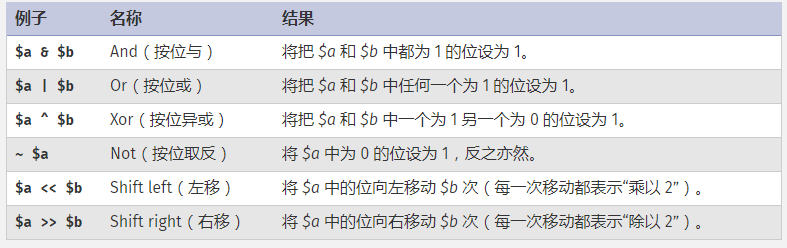#### 位运算及php错误报告设置

1 E_ERROR 0000 0001
2 E_WARNING 0000 0010
4 E_PARSE 0000 0100
8 E_NOTICE 0000 1000
###### 屏蔽错误提示
1. 在有可能出错的函数前加@,然后or die("")
@mysql_connect(...) or die("Database Connect Error")

1. 在php脚本前加error_reporting(0)，屏蔽所有错误提示。

## IP 域名及DNS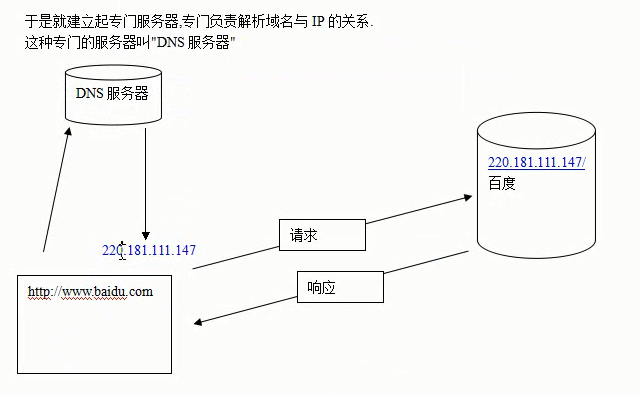## 文件引入

• include ""如果引入文件不存在，继续往下执行，报waring
• include_once
• require""引入文件不存在，包fatal error ,停止执行
• require_once

## Mysql入门

Mysql为关系型数据库

1. 连接到数据库服务器
mysql -h localhost -u root -p
2. 查看所有库
show databases;
3. 选库
use 库名
4. 查看库下的表
show tables;
5. 建表：
create table msg(
id int auto_increment primary key,
content varchar(200),
pubtime int
)charset utf8;
6. 告诉服务器我的字符集
set names utf8;
7. 添加数据
insert into msg(id,content,pubtime)values(1,‘逗你玩’,‘1111111’);
8. 查询所有数据
select * from msg;
9. 按id 查询
select * from msg where id=1;
10. 快速清空表
truncate 表名

## 浮点数不精确

if ((0.3 - 0.2) == 0.1) {
echo 'equal';
} else {
echo 'no equal';//no equal
}